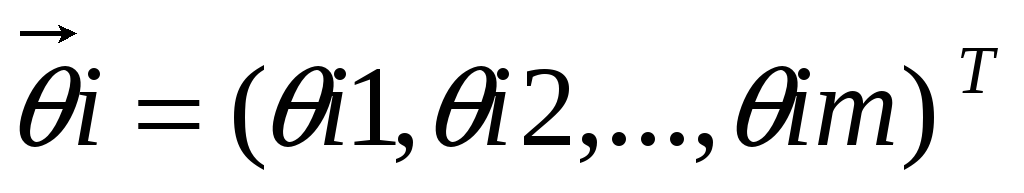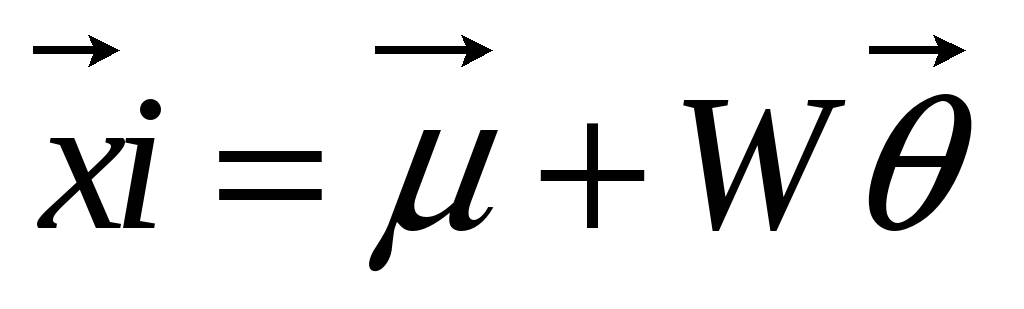# Chapter 5 1 5 1 Introduction 5

 Page 7/20 Date 23.12.2017 Size 0.6 Mb.

## Test and Train Sets

The data sets that have been used for the particular project are divided to two sub sets. The first is the training set that contains the images that were used in order to train the algorithm and the neural network. Training sets are used from the two training programs, arntrn and nntrn. Samples of the set can be found in appendix II. The other set is the other subset is the testing database, which contains different images than the training set but of the same people.

In MATLAB we use the commands imread and imresize in order to read the images and reduce the resolution. More detailed description of the commands and their properties is given in the code implementation chapter.

Chapter

5

## 5.1 Algorithms for face recognition

As mentioned in the introduction but also in other parts of the report, there are many algorithms that can be used for face recognition. Most of them are based on the same techniques and methods. Some of the most popular are Principal component analysis and the use of eigenfaces.

## 5.1.1 Principal Component Analysis

On the field of face recognition most of the common methods employ Principal Component Analysis. Principal Component Analysis is based on the Karhunen-Loeve (K-L), or Hostelling Transform, which is the optimal linear method for reducing redundancy, in the least mean squared reconstruction error sense. 1. PCA became popular for face recognition with the success of eigenfaces.

The idea of principal component analysis is based on the identification of linear transformation of the co-ordinates of a system. “The three axes of the new co-ordinate system coincide with the directions of the three largest spreads of the point distributions.”

In the new co-ordinate system that we have now the data is uncorrected with the data we had in the first co-ordinate system. 

For face recognition, given dataset of N training images, we create N d-dimensional vectors, where each pixel is a unique dimension. The principal components of this set of vectors is computed in order to obtain a d x m projection matrix, W. The image of the ith vector may be represented as weights:(1)Such that(2)

Approximates the original image where  is the mean, of the i and the reconstruction is perfect when m = d. P1

As mentioned before the ARENA algorithm is going to be tested and its performance is going to be compared with other algorithms. For the comparison we are going to use two different PCA algorithms. The first algorithm is computing and storing the weight of vectors for each person’s image in the training set, so the actual training data is not necessary. In the second algorithm each weight of each image is stored individually, is a memory-based algorithm. For that we need more storing space but the performance is better.

In order to implement the Principal component analysis in MATLAB we simply have to use the command prepca. The syntax of the command is

ptrans,transMat = prepca(P,min_frac)

Prepca pre-processes the network input training set by applying a principal component analysis. This analysis transforms the input data so that the elements of the input vector set will be uncorrected. In addition, the size of the input vectors may be reduced by retaining only those components, which contribute more than a specified fraction (min_frac) of the total variation in the data set.

Prepca takes these inputs the matrix of centred input (column) vectors, the minimum fraction variance component to keep and as result returns the transformed data set and the transformation matrix.

### 5.1.1.1 Algorithm

Principal component analysis uses singular value decomposition to compute the principal components. A matrix whose rows consist of the eigenvectors of the input covariance matrix multiplies the input vectors. This produces transformed input vectors whose components are uncorrected and ordered according to the magnitude of their variance.

Those components, which contribute only a small amount to the total variance in the data set, are eliminated. It is assumed that the input data set has already been normalised so that it has a zero mean.

In our test we are going to use two different “versions’ of PCA. In the first one the centroid of the weight vectors for each person’s images in the training set is computed and stored. On the other hand in PCA-2 a memory based variant of PCA, each of the weight vectors in individually computed and stored.